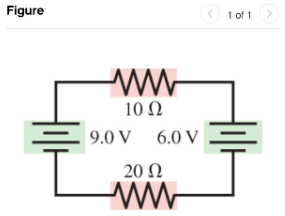# Problem: What are the magnitude and direction of the current in the 20 Ω resistor in (Figure 1)?

🤓 Based on our data, we think this question is relevant for Professor Kaplan's class at JHU.

###### FREE Expert Solution

Using Kirchhoff's loop rule in the clockwise direction:

$\overline{){\mathbf{\sum }}{\mathbf{V}}{\mathbf{=}}{\mathbf{0}}}$

$\begin{array}{rcl}\mathbf{9}\mathbf{.}\mathbf{0}\mathbf{V}\mathbf{-}& \mathbf{i}\mathbf{10}\mathbf{\Omega }\mathbf{-}\mathbf{6}\mathbf{.}\mathbf{0}\mathbf{V}\mathbf{-}\mathbf{i}\mathbf{20}\mathbf{\Omega }\mathbf{=}& \mathbf{0}\end{array}$###### Problem Details

What are the magnitude and direction of the current in the 20 Ω resistor in (Figure 1)?